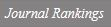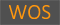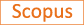An Evaluation of Weighted Chi-Square Statistics for Clustered Binary Data

Title
An Evaluation of Weighted Chi-Square Statistics for Clustered Binary Data
Authors
Ahn C.Jung S.-H.Kang S.-H.
Ewha Authors
Issue Date
2003
Journal Title
Drug Information Journal
ISSN
0092-8615Citation
Drug Information Journal vol. 37, no. 1, pp. 91 - 99
Indexed
SCOPUSDocument Type
Article
Abstract
Clustered binary responses occur frequently in many fields of application. Examples include the development of tumors in one or more animals of a litter, the presence of retinitis in either or both eyes of an AIDS patient, and spontaneous abortion of one or more implanted fetuses. When a binary response is observed in multiple units from each subject, application of the usual Pearson chi-square statistic is invalid since such responses within the same subject are not independent. In estimating the common response probability in clustered binary data, two weighting systems have been most popular: equal weights to units, and equal weights to clusters. We also include an optimal weighting method that minimizes the variance of the response probability. The weighted chi-square statistics using the above three weighting systems are applied to the real data arising from a teratologic study and an ophthalmologic study. We perform the simulation study to evaluate the performance of the three weighted chi-square statistics in terms of empirical type I errors and empirical powers. The simulation study shows that the weighted chi-square statistic using an optimal weight yields higher empirical powers than the other weighted chi-square statistics and produces empirical type I errors close to a nominal value. The wieghted chi-square statistics assigning equal weights to units (XD2) and optimal weights (XO2) are slightly anti-conservative when n1 = n2 = n = 10. We recommend using XO2 when n1 = n2 = n ≥ 20 since the differences in empirical type I errors are negligible among weighted chi-square statistics and XO2 performs better than the other weighted chi-square test statistics in empirical powers.
Appears in Collections:
자연과학대학 > 통계학전공 > Journal papers
Files in This Item:
There are no files associated with this item.
Export
RIS (EndNote)
XLS (Excel)
XML